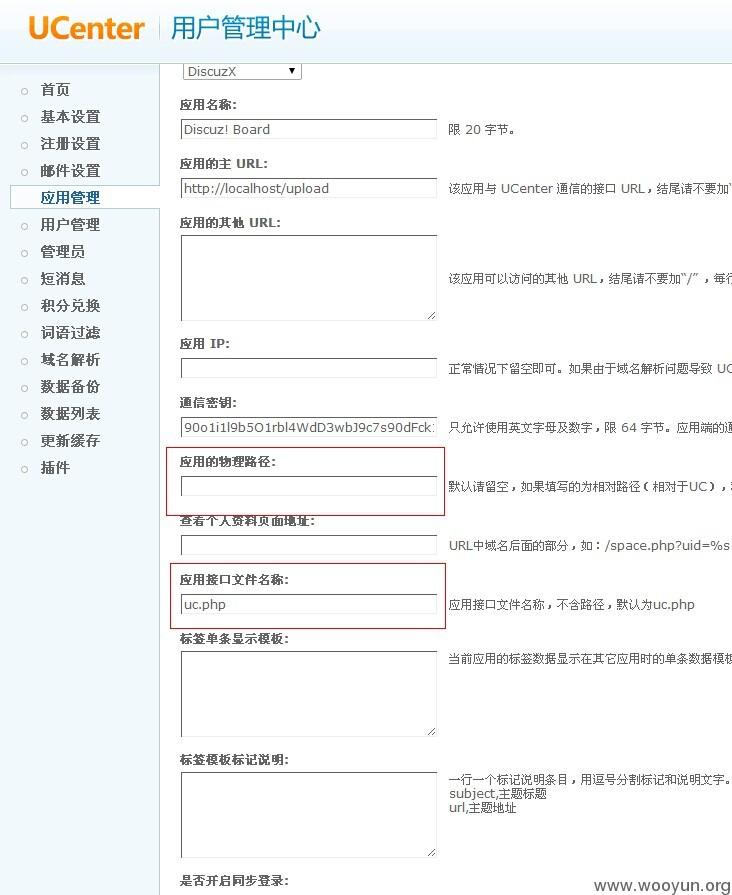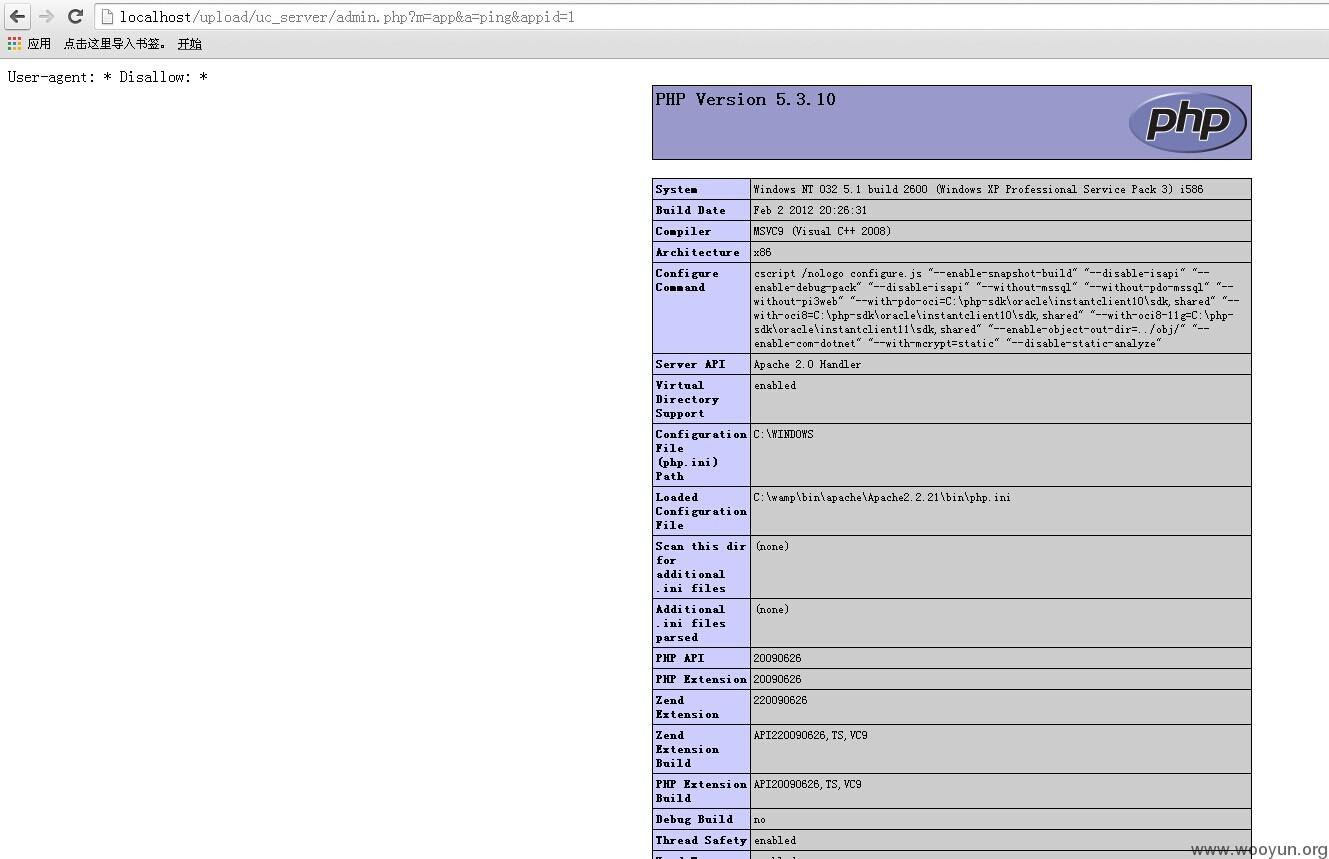## Discuz / DiscuzX .gitee-modal { width: 500px !important; }

Merged
!436 修复 UCenter任意文件包含Bug

function onping() {
$ip = getgpc('ip');$url = getgpc('url');
$appid = intval(getgpc('appid'));$app = $_ENV['app']->get_app_by_appid($appid);
$status = ''; if($app['extra']['apppath'] && @include $app['extra']['apppath'].'./api/'.$app['apifilename']) {
$uc_note = new uc_note();$status = $uc_note->test($note['getdata'], $note['postdata']); } else {$this->load('note');
$url =$_ENV['note']->get_url_code('test', '', $appid);$status = $_ENV['app']->test_api($url, $ip); } if($status == '1') {
echo 'document.getElementById(\'status_'.$appid.'\').innerHTML = "<img src=\'images/correct.gif\' border=\'0\' class=\'statimg\' \/><span class=\'green\'>'.$this->lang['app_connent_ok'].'</span>";testlink();';
} else {
echo 'document.getElementById(\'status_'.$appid.'\').innerHTML = "<img src=\'images/error.gif\' border=\'0\' class=\'statimg\' \/><span class=\'red\'>'.$this->lang['app_connent_false'].'</span>";testlink();';
}
}Show action logs Hide action logs
oldhuhu merged Pull Request 2020-04-14 15:02
oldhuhu test passed 2020-04-10 15:20
oldhuhu check passed 2020-04-10 15:20

PHP
1
https://gitee.com/Discuz/DiscuzX.git
git@gitee.com:Discuz/DiscuzX.git
Discuz
DiscuzX
DiscuzX